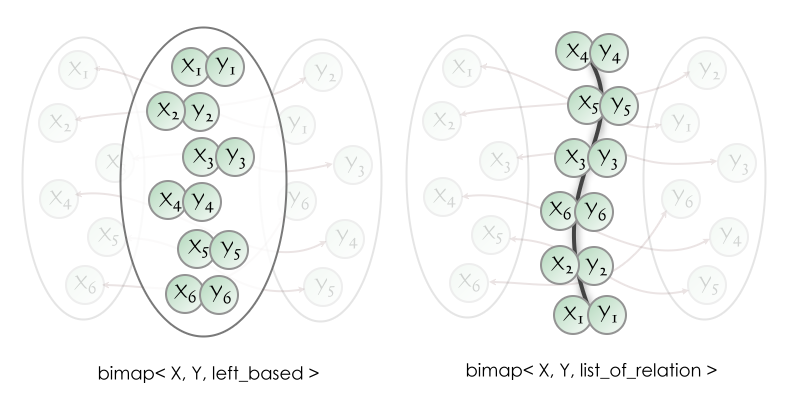#Boost C++ Libraries

...one of the most highly regarded and expertly designed C++ library projects in the world.

### The collection of relations type

A new point of view
Configuration parameters
Examples

#### A new point of view

Being able to change the collection type of the bimap relation view is another very important feature. Remember that this view allows the user to see the container as a group of the stored relations. This view has set semantics instead of map semantics.By default, Boost.Bimap will base the collection type of the relation on the type of the left collection. If the left collection type is a set, then the collection type of the relation will be a set with the same order as the left view.

In general, Boost.Bimap users will base the collection type of a relation on the type of the collection on one of the two sides. However there are times where it is useful to give this collection other constraints or simply to order it differently. The user is allowed to choose between:

• left_based
• right_based
• set_of_relation<>
• multiset_of_relation<>
• unordered_set_of_relation<>
• unordered_multiset_of_relation<>
• list_of_relation
• vector_of_relation
• unconstrained_set_of_relation
TipThe first two options and the last produce faster bimaps, so prefer these where possible.The collection type of relation can be used to create powerful containers. For example, if you need to maximize search speed, then the best bidirectional map possible is one that relates elements from an `unordered_set` to another `unordered_set`. The problem is that this container cannot be iterated. If you need to know the list of relations inside the container, you need another collection type of relation. In this case, a `list_of_relation` is a good choice. The resulting container trades insertion and deletion time against fast search capabilities and the possibility of bidirectional iteration.

```#include <iostream>
#include <string>
#include <boost/bimap/bimap.hpp>
#include <boost/bimap/list_of.hpp>
#include <boost/bimap/unordered_set_of.hpp>

struct english {};
struct spanish {};

int main()
{
using namespace boost::bimaps;

typedef bimap
<
unordered_set_of< tagged< std::string, spanish > >,
unordered_set_of< tagged< std::string, english > >,
list_of_relation

> translator;

translator trans;

// We have to use `push_back` because the collection of relations is
// a `list_of_relation`

trans.push_back( translator::value_type("hola"  ,"hello"   ) );
trans.push_back( translator::value_type("rosa"  ,"rose"    ) );
trans.push_back( translator::value_type("mesa"  ,"table"   ) );

std::cout << "enter a word" << std::endl;
std::string word;
std::getline(std::cin,word);

// Search the queried word on the from index (Spanish)

translator::map_by<spanish>::const_iterator is
= trans.by<spanish>().find(word);

if( is != trans.by<spanish>().end() )
{
std::cout << word << " is said "
<< is->get<english>()
<< " in English" << std::endl;
}
else
{

translator::map_by<english>::const_iterator ie
= trans.by<english>().find(word);

if( ie != trans.by<english>().end() )
{
std::cout << word << " is said "
<< ie->get<spanish>()
<< " in Spanish" << std::endl;
}
else
{

std::cout << "No such word in the dictionary"      << std::endl;
std::cout << "These are the possible translations" << std::endl;

for( translator::const_iterator
i = trans.begin(),
i_end = trans.end();

i != i_end ; ++i )
{
std::cout << i->get<spanish>()
<< " <---> "
<< i->get<english>()
<< std::endl;
}
}
}
return 0;
}
```

#### Configuration parameters

Each collection type of relation has different parameters to control its behaviour. For example, in the `set_of_relation` specification, you can pass a Functor type that compares two types. All of the parameters are exactly as in the standard library containers, except for the type, which is set to the bimap relation and the allocator type. To help users in the creation of each functor, the collection type of relation templates takes an mpl lambda expression where the relation type will be evaluated later. A placeholder named `_relation` is available to bimap users.

The following table lists the meaning of the parameters for each collection type of relations.

name

`left_based`

Not a template.

`right_based`

Not a template.

`set_of_relation<KeyComp>`

`multiset_of_relation<KeyComp>`

KeyComp is a Functor that compares two types using less than. By default, the less-than operator is `std::less<_relation>`.

`unordered_set_of_relation<HashFunctor,EqualKey>`

`unordered_multiset_of_relation<HashFunctor,EqualKey>`

HashFunctor converts the `relation` into an `std::size_t` value. By default it is `boost::hash<_relation>`.

EqualKey is a Functor that tests two relations for equality. By default, the equality operator is `std::equal_to<_relation>`.

`list_of_relation`

Not a template.

`vector_of_relation`

Not a template.

`unconstrained_set_of_relation`

Not a template.

#### Examples

Consider this example:

```template< class Rel >
struct RelOrder
{
bool operator()(Rel ra, Rel rb) const
{
return (ra.left+ra.right) < (rb.left+rb.right);
}
};

typedef bimap
<
multiset_of< int >,
multiset_of< int >,
set_of_relation< RelOrder<_relation> >

> bimap_type;
```

Here the bimap relation view is ordered using the information of both sides. This container will only allow unique relations because `set_of_relation` has been used but the elements in each side of the bimap can be repeated.

```struct name         {};
struct phone_number {};

typedef bimap
<
tagged< unordered_multiset_of< string >, name         >,
tagged< unordered_set_of     < int    >, phone_number >,
set_of_relation<>

> bimap_type;
```

In this other case the bimap will relate names to phone numbers. Names can be repeated and phone numbers are unique. You can perform quick searches by name or phone number and the container can be viewed ordered using the relation view.